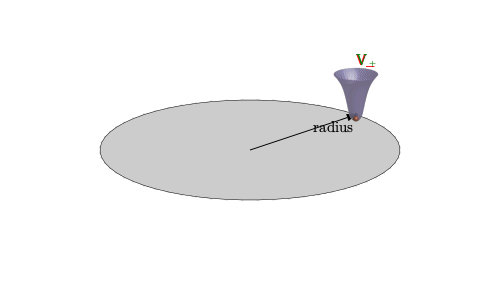# Recommendation for animated 3D diagram

I’m contemplating trying to make an animation based on the following figure:

In the animation, I’d like to have the two red cups (and the blue balls contained in them) rotate independently around the circle, with a position ϕ(t) that follows a specific equation.

Does anyone have a recommendation on what packages to use to create such an animation? It doesn’t need to copy the figure exactly, but I’d like to more or less retain the 3D-like character, and the unicode labels are pretty important. It doesn’t need to be fancy 3D (raytraced). In fact, I’d probably prefer the “diagram” look that the figure has now.

I’m open to any solutions in Julia or Python (matplotlib), and possibly also TeX/TikZ in combination with some scripting (this animation is going into a Beamer presentation). If someone has code-snippets for parts of such a figure, to get me started, that would be especially helpful, since I’d need to finish this animation within the next 24 hours or so if I want to put it in the talk.(the original was created by a collaborator, probably mostly manually in Inkscape; I don’t have access to the underlying document)

2 Likes

I’m making some progress with TikZ based on 3d-plane and rotating coordinate system in TiKZ - TeX - LaTeX Stack Exchange and having been able to extract just the “cup” from the figure as a semi-transparent pdf, so I think that’s going to be the way to go…

I defined a “bell” as minus the rescaled pdf of the bivariate normal distribution and this is how it looks: https://nbviewer.org/gist/empet/8879dbaecd6f46e4ebcb2526ec8c0df0. The perspective projection makes the two “bells” look as being non-orthogonal to the disk. Also the lighting could be better.

2 Likes

I expect Makie can do this.

1 Like

Well, TikZ with a little bit of Python scripting did the trick:

(source once I clean this up and put it on Github later this week)

3 Likes

I’ll be waiting for the source code for this in Tikz Latex. No Julia expert here can create animation for this one?

1 Like

Here’s a sketch with Makie:

``````f(x, y) = 1-exp(-(x^2+y^2))

r = 0:0.1:2
theta = LinRange(-pi, pi, 100)
x = r .* cos.(theta')
y = r .* sin.(theta')

R = 5.0
alpha = Observable(0.0)
x0 = @lift(R*cos(\$alpha))
y0 = @lift(R*sin(\$alpha))

fig = Figure()
ax = Axis3(fig[1,1], aspect=(3,3,1))
surface!(ax, @lift(x .- \$x0), @lift(y .- \$y0), f.(x, y), color=fill(:blue, size(x)), transparency=true)
surface!(ax, @lift(x .- \$x0), @lift(y .+ \$y0), f.(x, y), color=fill(:red, size(x)), transparency=true)
lines!(ax, R*cos.(theta), R*sin.(theta), color=:black, linestyle=:dash)
hidedecorations!(ax)
hidespines!(ax)

record(fig, "figure.mp4", -pi:0.05:pi; framerate=30) do α
alpha[] = α
end
``````

6 Likes

This reminds me of an old question of mine on stackexchange … pgfplots - How to display a duck or marmot swallowed by a darkhole - TeX - LaTeX Stack Exchange

A bit late - I hope your talk went well!

This was a little challenging in Julia, I admit …3 Likes

Ok, so the Python/TikZ source for the animation is now at

(together with a call to `ffmpeg` in the `Makefile`)

A bit late - I hope your talk went well!

Thanks! I think so!A recording of the trial talk is on YouTube, for anyone who might be interested.

Even though TikZ turned out as the easiest way for me to make this animation, I’d like to thank everyone for their suggestions! It’s definitely good to have some ideas on how to do these kinds of animation with Julia.

Is that done with `Luxor.jl` (which is definitely a package I’d like to explore for this kind of thing, alongside Makie)? Can you share the script you used to generate the animation?

haha, sure, I just wanted to see how tricky it would be… (I needed some 3D points at one stage…)

1 Like